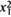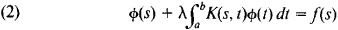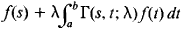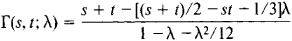# Resolvent

(redirected from resolvents)
Also found in: Dictionary, Thesaurus, Medical.

## resolvent

[ri′zäl·vənt]
(mathematics)
For a linear operator T on a Banach space, the function, defined on the complement of the spectrum of T given by (T- λ I)-1 for each λ in this complement, where I is the identity operator; this enables a study of T relative to its eigenvalues.

## Resolvent

a mathematical term with various meanings. We speak, for example, of resolvent equations, resolvent kernels, and resolvent operators.

In algebra, the term “resolvent” is used in several senses. Thus, by the resolvent of the algebraic equation f(x) = 0 of degree n we mean an algebraic equation g(x) = 0 such that its coefficients are rational functions of the coefficients of f(x) and, if the roots of this equation are known, it is possible to find the roots of f(x) = 0 by solving simpler equations of degree at most n. For example, the equation

ν3 - a2v2 + (a1a3 - 4α4) ν - (α\αΛ - 2 αΛ + a]) = 0

is one of the cubic resolvents of the fourth-degree equation

(1) x4 + a1x3 + a2x2 + a3x + a4 = 0

If ν1, v2, and v3 are the roots of the resolvent equation, the roots x1, x2, x3, and x4 of equation (1) can be found by solving the quadratic equations σ2 - vk σ + a4 = 0, k = 1, 2, 3. Thus, if xn and nk are the roots of these quadratic equations, x1x2= x3x4 = n, x1x3 = x2x2x4 = x2x2x4 = n3,= ξ1x2/n3, and so on. A Galois resolvent of the equation f(x) is an algebraic equation g(x) = 0 irreducible over a given field (seeGALOIS THEORY) such that when one of its roots is adjoined to the field, there results a field containing all the roots of the equation f(x) = 0.

The term “resolvent” is used in a somewhat different sense in what is known as the Hilbert-Chebotarev resolvent problem.

In the theory of integral equations, the resolvent of the equationis a function Γ (s, t; λ) of the variables s and t and the parameter λ such that the solution of equation (2) can be represented in the formprovided that λ is not an eigenvalue of equation (2). For example, the resolvent of the kernel K(s, t) = S + t is the functionIn the theory of linear operators, the resolvent of the operator A is the family of operators Rλ = (A - λ E)-1 where the complex parameter λ takes on any values outside the spectrum of A.

References in periodicals archive ?
It is bounded, continuous, and maximal monotone such that [mathematical expression not reproducible], is called the "Yosida resolvent" of T.
(iv) [f.sup.*] [member of] (T+C)(D(T)[intersection] [B.sub.R](0)) ifT is of compact resolvent and, C is bounded and continuous.
A resolution chain [C.sub.1], [C.sub.2], ..., [C.sub.n] is called a linear regular resolution chain if there exists a sequence of clauses [D.sub.1], [D.sub.2], ..., [D.sub.n-1] such that: (1) [D.sub.1] is the resolvent of [C.sub.1] and [C.sub.2]; (2) for 2 [less than or equal to] i < n, [D.sub.1] is the resolvent of [D.sub.i-1] and [C.sub.i+1]; (3) all resolutions are applied on different T-atoms.
Usually, C' [disjunction] l' is a resolvent of a linear resolution.
now since [sub.2]M and [sub.4]M are the same literal M and the index 2 < index 4, so the resolvent of (1) and (2) will be (4) and is called a lock resolvent of clause (1) and clause (2).
The value of the norm of the resolvent f depends in particular on how close the eigenvalues of A are to the closed negative real axis.
In [32,33], the authors established the general theory of fractional resolvent and studied its application to the well posed problem for the evolution equation below:
see , , ,  for more properties of the resolvent.
This will be based on two conceptual pillars: (a) the actual numerical realization of projections of the form [Q.sub.[alpha]] as well as of [e.sup.-tA] within any desired target accuracy; (b) the involved application of resolvents [([gamma]I - A).sup.-1] appearing in the Dunford integral is based on adaptive wavelet schemes again realizing proper target accuracies without ever fixing any discretization of A beforehand.
Polynomial numerical hulls also can be used to gain information about the resolvent norm, [parallel][([zeta]I - A).sup.-1][parallel], for values of [zeta] throughout the complex plane.
means that p (X) can be selected in a resolvent c, Q only if c forces X to assume values greater than 0.
By Lemma 3, a resolvent of a maximal monotone operator is firmly nonexpansive.

Site: Follow: Share:
Open / Close